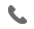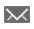•+44 7459 302492info@uplatz.com
• Register
Job Meter = High

### Advanced Statistical Analysis Using IBM SPSS Statistics (V26)

30 Hours
Online Instructor-led Training
USD 1399 (USD 2800)
Save 50% Offer ends on 31-Dec-2023214 Learners

This course provides an application-oriented introduction to advanced statistical methods available in IBM SPSS Statistics. Students will review a variety of advanced statistical techniques and discuss situations in which each technique would be used, the assumptions made by each method, how to set up the analysis, and how to interpret the results. This includes a broad range of techniques for predicting variables, as well as methods to cluster variables and cases.

-----------------------------------------------------------------------------------

Course Objective

• Introduction to advanced statistical analysis

• Grouping variables with Factor Analysis and Principal Components Analysis

• Grouping cases with Cluster Analysis

• Predicting categorical targets with Nearest Neighbor Analysis

• Predicting categorical targets with Discriminant Analysis

• Predicting categorical targets with Logistic Regression

• Predicting categorical targets with Decision Trees

• Introduction to Survival Analysis

• Introduction to Generalized Linear Models

• Introduction to Linear Mixed

• Models

-----------------------------------------------------------------------------------

Course Description

Advanced Statistical Analysis using IBM SPSS Modeler V18.1.1 online course get started with fundamentals of statistical techniques. Advanced Statistical Analysis using IBM SPSS Modeler V18.1.1 online course intention is to provide a foundation to implement advanced statistical techniques.

Advanced Statistical Analysis using IBM SPSS Modeler V18.1.1 online course will allow the participants to understand the benefits of learning familiarity with modeler environment.

Advanced Statistical Analysis using IBM SPSS Modeler V18.1.1 online course is ideally developed for any professionals who wants to expertise in advanced statistical methods.

In the Advanced Statistical Analysis using IBM SPSS Modeler V18.1.1 online training course, Uplatz provides an in-depth online training for the participants or learners to gain knowledge and able to use components of IBM SPSS modeler. Uplatz provides appropriate teaching and expertise training to equip the participants for implementing the learnt concepts in an enterprise.

Advanced Statistical Analysis using IBM SPSS Modeler V18.1.1 online training course curriculum covers introduction to advanced statistical analysis, group variables using factor analysis, group cases with cluster analysis.

With the help of Advanced Statistical Analysis using IBM SPSS Modeler V18.1.1 online course, the learners can discover:

• Inhouse Terminology and concepts related to the Advanced Statistical Analysis using IBM SPSS Modeler V18.1.1

• Learn how to use survival analysis

• Implement modeler strategy

• Accelerate model performance

Uplatz provides an in-depth training to the learners to accelerate their knowledge and skill set required for a statistical modeler

-----------------------------------------------------------------------------------

# Advanced Statistical Analysis Using IBM SPSS Statistics (V26)

Course Details & Curriculum
• Taxonomy of models
• Overview of supervised models
• Overview of models to create natural groupings

Grouping variables with Factor Analysis and Principal Components Analysis
• Factor Analysis basics
• Principal Components basics
• Assumptions of Factor Analysis
• Key issues in Factor Analysis
• Use Factor and component scores

Grouping cases with Cluster Analysis
• Cluster Analysis basics
• Key issues in Cluster Analysis
• K-Means Cluster Analysis
• Assumptions of K-Means Cluster Analysis
• TwoStep Cluster Analysis
• Assumptions of TwoStep Cluster Analysis

Predicting categorical targets with Nearest Neighbor Analysis
• Nearest Neighbors Analysis basics
• Key issues in Nearest Neighbor Analysis
• Assess model fit

Predicting categorical targets with Discriminant Analysis
• Discriminant Analysis basics
• The Discriminant Analysis model
• Assumptions of Discriminant Analysis
• Validate the solution

Predicting categorical targets with Logistic Regression
• Binary Logistic Regression basics
• The Binary Logistic Regression model
• Multinomial Logistic Regression basics
• Assumptions of Logistic Regression procedures
• Test hypotheses
• ROC curves

Predicting categorical targets with Decision Trees
• Decision Trees basics
• Explore CHAID
• Explore C&RT
• Compare Decision Trees methods

Introduction to Survival Analysis
• Survival Analysis basics
• Kaplan-Meier Analysis
• Assumptions of Kaplan-Meier Analysis
• Cox Regression
• Assumptions of Cox Regression

Introduction to Generalized Linear Models
• Generalized Linear Models basics
• Available distributions

Introduction to Linear Mixed Models
• Linear Mixed Models basics
• Hierarchical Linear Models
• Modeling strategy
• Assumptions of Linear Mixed Models
-----------------------------------------------------------------------------------
Career Path

Advanced Statistical Analysis using IBM SPSS Modeler V18.1.1 online certification course with the help of expert professionals training is recognized across the globe. Because of the increased adoption of the statistical analysis concepts in various companies the participants are able to find the job opportunity easily. The leading companies hire statistical modeler considering their skill of mastering every ground aspect related to its efficiency and advanced statistical performance. Advanced Statistical Analysis using IBM SPSS Modeler V18.1.1 online certification course is known for their knowledge in implementing statistical techniques. After pursuing Advanced Statistical Analysis using IBM SPSS Modeler V18.1.1 online certification course the participants can become as a data statistics expert, IBM modeler, SPSS modeler and can pursue a wide range of career paths.
-----------------------------------------------------------------------------------